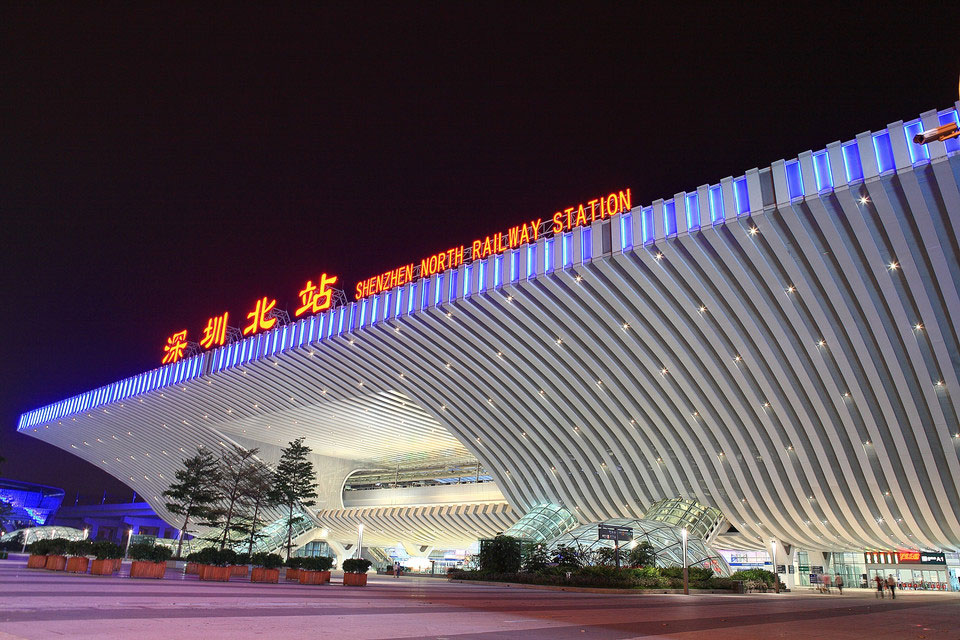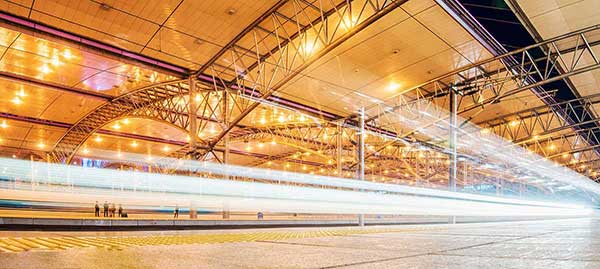<tr id="6c00a"><xmp id="6c00a">

•廣州南站

可用休息室6個

可用餐廳3個

•深圳北站

可用休息室6個

可用餐廳0個

•長沙南站

可用休息室2個

可用餐廳1個

•重慶北站

可用休息室1個

可用餐廳0個

•西安北站

可用休息室1個

可用餐廳0個

•沈陽北站

可用休息室2個

可用餐廳0個

• 高鐵列表
• 全部地點 中國大陸 其他地區

A

• 奧倫堡火車站俄羅斯聯邦

B

• 北京南站

• 北京西站

• 北京站

• 璧山站

C

• 重慶北站

HOT
• 成都東站

• 長沙南站

HOT
• 長春西站

• 重慶西站

• 車里雅賓斯火車站俄羅斯聯邦

D

• 大連北站

• 大連站

• 墊江站

• 大足南站

• 東京站日本

E

• 鄂木斯克火車站俄羅斯聯邦

F

• 福州南站

• 福州站

• 福岡高鐵博多站日本

G

• 廣州南站

HOT
• 貴陽北站

• 桂林北站

• 桂林站

H

• 哈爾濱西站

• 杭州東站

• 合井站韓國

• 合肥站

• 合肥南站

• 杭州站

• 漢口站

J

• 簡陽南站

• 濟南西站

K

• 京都站日本

• 昆明南站

L

• 隆昌北站

• 蘭州西站

• 羅馬特米尼火車站意大利

• 柳州站

• 梁平南站

M

• 明洞站韓國

N

• 南寧站

• 南昌火車站

• 南昌西站

• 內江北站

• 寧波站

• 南京南站

• 南寧東站

• 南京站

O

• 大阪高鐵站日本

Q

• 泉州站

• 青島北站

• 秋明火車站俄羅斯聯邦

R

• 榮昌北站

S

• 三亞站

• 深圳北站

HOT
• 上海站

• 沈陽北站

HOT
• 石家莊站

• 首爾站韓國

• 蘇州北站

• 蘇州站

• 上海虹橋站

• 沈陽站

• 上海西站

T

• 天津南站

• 天津西站

• 太原站

• 太原南站

• 唐山站

W

• 武漢站

• 溫州南站

• 無錫站

• 無錫東站

• 無錫新區站

• 烏蘭烏德火車站俄羅斯聯邦

• 萬州北站

X

• 西安北站

HOT
• 廈門站

• 徐州東站

• 廈門北站

• 新西伯利亞火車站俄羅斯聯邦

Y

• 永川東站

• 鹽城站

• 伊爾庫茨克火車站俄羅斯聯邦

• 葉卡捷琳堡火車站俄羅斯聯邦

Z

• 珠海站

• 資陽北站

• 鄭州東站

• 資中北站

A

B

C

D

E

F

G

H

J

K

L

M

N

O

Q

R

S

T

W

X

Y

Z